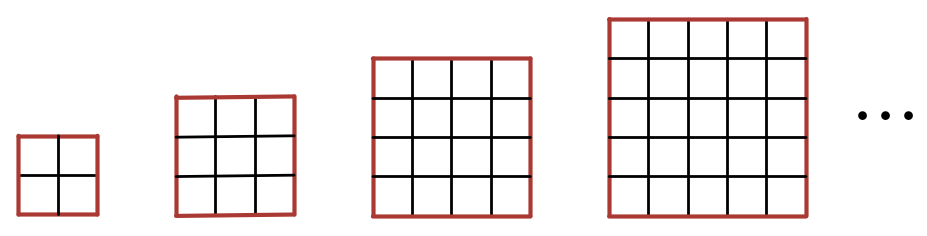# Red Perimeter 2 – Express With Variables

Probability Level 1Which of the following expresses the correct formula for the number of unit squares with exactly 1 red side in an $n \times n$ square with $n \geq 2$ ?

×